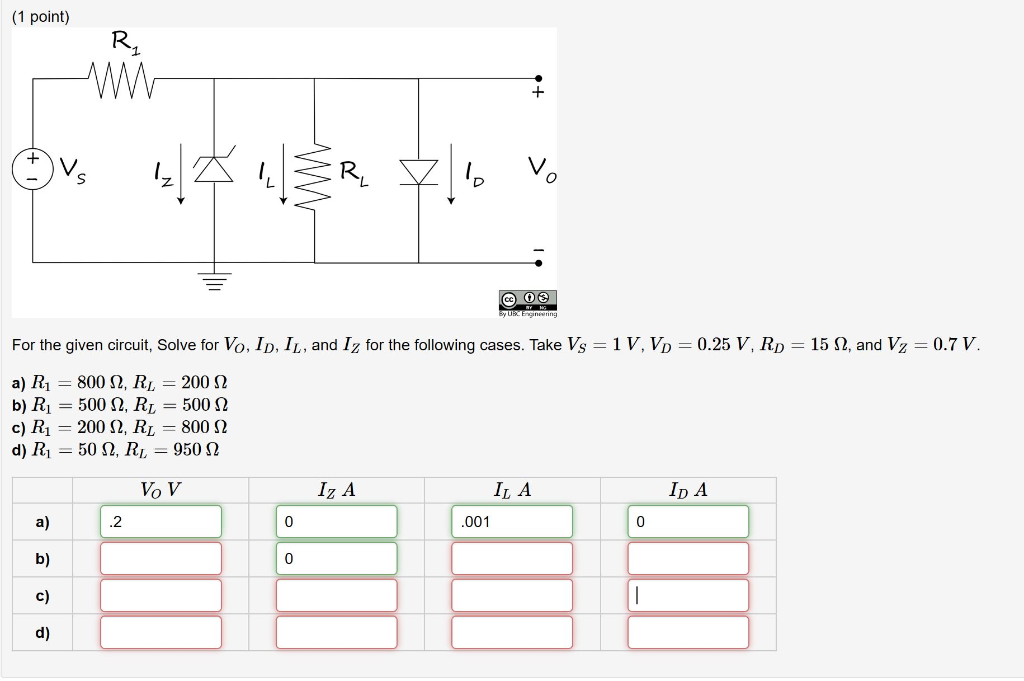# Question For the given circuit, Solve for VO , ID , IL , and IZ for thefollowing cases. Take VS=1 V , VD=0.25 V , RD=15Ω , and VZ=0.7 V.a) R1=800 Ω , RL=200 Ωb) R1=500Ω , RL=500Ωc) R1=200Ω ,RL=800Ωd) R1=50Ω ,RL=950Ω (1 point) Ov: 141 ŠR lo vo @ 0 For the given circuit, Solve for Vo, ID, IL, and Iz for the following cases. Take Vs = 1V, Vp = 0.25 V, Rp = 15 12, and V2 = 0.7 V. a) Ri = 800 12, R = 200 12 b) R1 = 500 12, RL = 500 12 c) R1 = 200 S2, RL = 800 32 d) R1 = 502, RL = 950 22 VOV Iz A ILA Ip A .001CEGT86 The Asker · Electrical Engineering

For the given circuit, Solve for VO , ID , IL , and IZ for the following cases. Take VS=1 V , VD=0.25 V , RD=15Ω , and VZ=0.7 V .a) R1=800 Ω , RL=200 Ω
b) R1=500Ω , RL=500 Ω
c) R1=200Ω , RL=800Ω
d) R1=50Ω , RL=950Ω

Transcribed Image Text: (1 point) Ov: 141 ŠR lo vo @ 0 For the given circuit, Solve for Vo, ID, IL, and Iz for the following cases. Take Vs = 1V, Vp = 0.25 V, Rp = 15 12, and V2 = 0.7 V. a) Ri = 800 12, R = 200 12 b) R1 = 500 12, RL = 500 12 c) R1 = 200 S2, RL = 800 32 d) R1 = 502, RL = 950 22 VOV Iz A ILA Ip A .001
More
Transcribed Image Text: (1 point) Ov: 141 ŠR lo vo @ 0 For the given circuit, Solve for Vo, ID, IL, and Iz for the following cases. Take Vs = 1V, Vp = 0.25 V, Rp = 15 12, and V2 = 0.7 V. a) Ri = 800 12, R = 200 12 b) R1 = 500 12, RL = 500 12 c) R1 = 200 S2, RL = 800 32 d) R1 = 502, RL = 950 22 VOV Iz A ILA Ip A .001